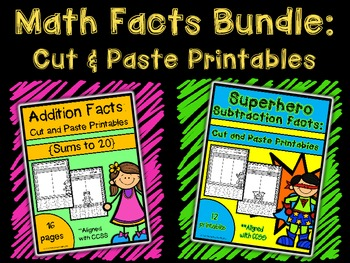# Math Facts Bundle: Cut and Paste Printables {Addition & Subtraction}Subject
Resource Type
Format
PDF (7 MB|33 pages)
Standards
\$8.00
List Price:
\$10.00
You Save:
\$2.00
\$8.00
List Price:
\$10.00
You Save:
\$2.00

### Description

This Math bundle includes both my Addition Facts and Superhero Subtraction Facts Cut and Paste Printables. I have bundled these products in order to offer them at a discounted price if purchased together.

Addition Facts: Cut and Paste Printables {Sums to 20}
--This set of 16 printables provide hands-on practice of addition facts with sums to 20. The cut and paste sorting format of these printables are sure to get students engaged therefore ensuring better fluency of basic math facts. By purchasing this set you will receive 16 printable activities filled with sorting activities of varying degrees of difficulty.

Superhero Subtraction Facts: Cut and Paste Printables
--This set of 12 printables provide hands-on practice of subtraction facts. The cut and paste sorting format of these printables are sure to engage students therefore ensuring better fluency of basic math facts. By purchasing this set you will receive 12 printable activities filled with sorting activities at varying degrees of difficulty.

** Download the preview to see a snapshot of all pages included. All activities are aligned with Common Core State Standards! Thanks for stopping by my store. Feel free to contact me with any product suggestions, comments, or questions. My email address is morethanmath@yahoo.com.
Total Pages
33 pages
N/A
Teaching Duration
N/A
Report this Resource to TpT
Reported resources will be reviewed by our team. Report this resource to let us know if this resource violates TpT’s content guidelines.

### Standards

to see state-specific standards (only available in the US).
Fluently add and subtract within 20 using mental strategies. By end of Grade 2, know from memory all sums of two one-digit numbers.
Understand the meaning of the equal sign, and determine if equations involving addition and subtraction are true or false. For example, which of the following equations are true and which are false? 6 = 6, 7 = 8 - 1, 5 + 2 = 2 + 5, 4 + 1 = 5 + 2.
Add and subtract within 20, demonstrating fluency for addition and subtraction within 10. Use strategies such as counting on; making ten (e.g., 8 + 6 = 8 + 2 + 4 = 10 + 4 = 14); decomposing a number leading to a ten (e.g., 13 - 4 = 13 - 3 - 1 = 10 - 1 = 9); using the relationship between addition and subtraction (e.g., knowing that 8 + 4 = 12, one knows 12 - 8 = 4); and creating equivalent but easier or known sums (e.g., adding 6 + 7 by creating the known equivalent 6 + 6 + 1 = 12 + 1 = 13).